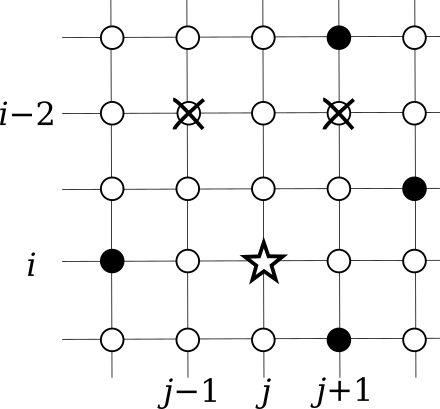시간 제한 메모리 제한 제출 정답 맞은 사람 정답 비율
2 초 512 MB 0 0 0 0.000%

## 문제

You are given a set of points aligned in a grid of N rows and M columns. Each of the points is named Pij with its row number i (1 ≤ i ≤ N) and its column number j (1 ≤ j ≤ M).

Each point is colored either white or black. Your task is to compute the number of subsets T of white points satisfying the following conditions:

• i ∈ {3, 4,..., N}, ∀j ∈ {2, 3,..., M}, Pij ∈ T ⇒ Pi -2, j -1 ∉ T,
• i ∈ {3, 4,..., N}, ∀j ∈ {1, 2,..., M−1}, Pij ∈ T ⇒ Pi -2, j +1 ∉ T.

For example, in the figure below, if you include the point marked with a star to the set T, you can include neither of the two crossed points.If you are familiar with the game of shogi (Japanese chess), you may find similarities between the possible moves of a keima with the relative positions of the star point and crossed points.

Because the answer can be huge, output the remainder divided by 109+7.

## 입력

The input consists of multiple datasets, each in the following format.

N M a1,1 ... a1,M ... aN,1 ... aN,M

The first line of each dataset contains two integers N (2 ≤ N ≤ 60) and M (2 ≤ M ≤ 60) representing the numbers of rows and columns of the grid, respectively. Each of the following N lines contains M characters. The j-th character of the i-th line, ai,j, represents the color of the point Pi, j, where '.' denotes white and '#' denotes black.

The end of the input is indicated by a line containing two zeros.

The number of datasets does not exceed 100.

## 출력

For each dataset, output one line containing the number of subsets satisfying the conditions modulo 109+7.

## 예제 입력 1

3 2
.#
##
#.
3 3
.#.
#.#
..#
6 5
.#..#
...#.
#....
..#.#
#....
..#..
0 0


## 예제 출력 1

3
20
103320